# Polygon Tiling Examples

Uniform tilings

Non-uniform tilings

Several uniform regular polygon tiling patterns can be achieved by use of grid.pattern_regular_polygon() plus occasionally grid.polygon() to set a background color. This vignette highlights several such tiling patterns plus a couple notable non-uniform tiling patterns.

library("grid")
library("gridpattern")
pushViewport(viewport(x=0.25, y=0.75, width=0.5, height=0.5))
grid.rect(gp = gpar(fill = NA, col = "white", lwd = 6))
popViewport()
pushViewport(viewport(x=0.75, y=0.75, width=0.5, height=0.5))
grid.rect(gp = gpar(fill = NA, col = "white", lwd = 6))
popViewport()
pushViewport(viewport(x=0.25, y=0.25, width=0.5, height=0.5))
grid.rect(gp = gpar(fill = NA, col = "white", lwd = 6))
popViewport()
pushViewport(viewport(x=0.75, y=0.25, width=0.5, height=0.5))
grid.rect(gp = gpar(fill = NA, col = "white", lwd = 6))
popViewport()
}
x_sq <- c(0, 0, 1, 1)
y_sq <- c(0, 1, 1, 0)
# colorblind accessible scheme https://jfly.uni-koeln.de/color/
blue <- grDevices::rgb(0.35, 0.70, 0.90)
yellow <- grDevices::rgb(0.95, 0.90, 0.25)
red <- grDevices::rgb(0.80, 0.40, 0.00)
green <- grDevices::rgb(0.00, 0.60, 0.50)
orange <- grDevices::rgb(0.90, 0.60, 0.00)

# Uniform tilings

## Triangular tiling

When we consider the background to be a triangle fill color we can straight-forwardly achieve several of the uniform colorings of a triangular tiling using the “convex3” shape with the “hex” grid.

grid.triangular_tiling <- function(...) {
grid.pattern_regular_polygon(..., shape = "convex3", density = 1, grid = "hex",
spacing = 0.2, angle = 0, rot = 180)
}
# 1 color uniform triangular tiling
pushViewport(viewport(x=0.25, y=0.75, width=0.5, height=0.5))
grid.polygon(x_sq, y_sq, gp = gpar(fill = yellow, col = NA))
gp <- gpar(fill = yellow, col = "black")
grid.triangular_tiling(gp = gp)
popViewport()

# 2 color uniform triangular tiling
pushViewport(viewport(x=0.75, y=0.75, width=0.5, height=0.5))
grid.polygon(x_sq, y_sq, gp = gpar(fill = yellow, col = NA))
gp <- gpar(fill = red, col = "black")
grid.triangular_tiling(gp = gp)
popViewport()

# 3 color uniform triangular tiling
pushViewport(viewport(x=0.25, y=0.25, width=0.5, height=0.5))
grid.polygon(x_sq, y_sq, gp = gpar(fill = yellow, col = NA))
gp <- gpar(fill = c(blue, red), col = "black")
grid.triangular_tiling(gp = gp)
popViewport()

# 4 color uniform triangular tiling
pushViewport(viewport(x=0.75, y=0.25, width=0.5, height=0.5))
grid.polygon(x_sq, y_sq, gp = gpar(fill = yellow, col = NA))
gp <- gpar(fill = c(red, blue, green), col = "black")
grid.triangular_tiling(gp = gp)
popViewport()
add_borders()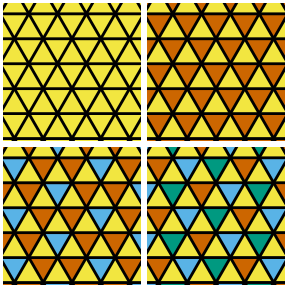## Square tiling

We can achieve the nine non-staggered uniform colorings of a square tiling using the “square” shape and the appropriate “type” (and perhaps “subtype”).

# 2 color uniform square tiling (1212)
pushViewport(viewport(x=0.25, y=0.75, width=0.5, height=0.5))
gp <- gpar(fill = c(yellow, red), col = "black")
grid.pattern_regular_polygon(shape = "square", density = 1,
spacing = 0.2, angle = 0, gp = gp)
popViewport()
# 2 color uniform square tiling (1122)
pushViewport(viewport(x=0.75, y=0.75, width=0.5, height=0.5))
gp <- gpar(fill = c(yellow, red), col = "black")
grid.pattern_regular_polygon(shape = "square", density = 1, type = "horizontal",
spacing = 0.2, angle = 0, gp = gp)
popViewport()
# 3 color uniform square tiling (1213)
pushViewport(viewport(x=0.25, y=0.25, width=0.5, height=0.5))
gp <- gpar(fill = c(yellow, red, blue), col = "black")
grid.pattern_regular_polygon(shape = "square", density = 1,
spacing = 0.2, angle = 0, gp = gp)
popViewport()
# 4 color uniform square tiling (1234)
pushViewport(viewport(x=0.75, y=0.25, width=0.5, height=0.5))
gp <- gpar(fill = c(yellow, red, blue, green), col = "black")
grid.pattern_regular_polygon(shape = "square", density = 1,
spacing = 0.2, angle = 0, gp = gp)
popViewport()
add_borders()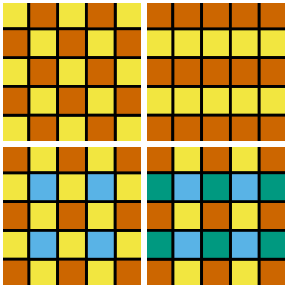## Hexagonal tiling

We can achieve all the uniform colorings of a hexagonal tiling using the “convex6” shape with the “hex” grid and the appropriate “type”. Replacing the “convex6” shape with an appropriately scaled “star6” shape results in a uniform tiling using star polygons.

grid.hexagonal_tiling <- function(..., shape = "convex6", spacing = 0.2) {
scale <- star_scale(6, 30)
grid.pattern_regular_polygon(..., shape = shape, density = 1, grid = "hex",
spacing = spacing, scale = scale, angle = 0)
}
# 1 color uniform hexagonal tiling
pushViewport(viewport(x=0.25, y=0.75, width=0.5, height=0.5))
gp <- gpar(fill = yellow, col = "black")
grid.hexagonal_tiling(gp = gp)
popViewport()

# One of several 2 color uniform hexagonal tiling
pushViewport(viewport(x=0.75, y=0.75, width=0.5, height=0.5))
gp <- gpar(fill = c(red, yellow), col = "black")
grid.hexagonal_tiling(gp = gp)
popViewport()

# 3 color uniform hexagonal tiling
pushViewport(viewport(x=0.25, y=0.25, width=0.5, height=0.5))
gp <- gpar(fill = c(yellow, red, blue), col = "black")
grid.hexagonal_tiling(gp = gp)
popViewport()

# (4.6_{π/6})^3
pushViewport(viewport(x=0.75, y=0.25, width=0.5, height=0.5))
grid.polygon(x_sq, y_sq, gp = gpar(fill = yellow, col = NA))
gp <- gpar(fill = red, col = "black")
grid.hexagonal_tiling(shape = "star6", spacing = 0.3, gp = gp)
popViewport()
add_borders()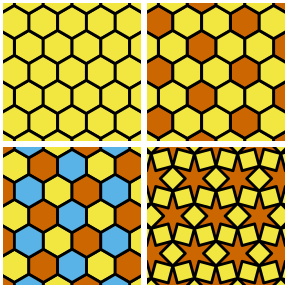## Snub square tiling

We can achieve the two uniform colorings of a snub square tiling using a “star4” shape and a “convex4” shape.

grid.snub_square <- function(..., gp_sq, gp_sq2 = gp_sq, gp_tri = gp_sq) {
scale_star <- star_scale(4, 90 + 60, external = TRUE)
grid.polygon(x_sq, y_sq, gp = gp_sq)
grid.pattern_regular_polygon(shape = "star4", scale = scale_star,
angle = 0, rot = 15, spacing = 0.2,
density = 1.4, gp = gp_tri)
grid.pattern_regular_polygon(shape = "convex4", scale = scale_star,
angle = 0, rot = 60, spacing = 0.2,
density = scale_star * 1.4, gp = gp_sq2)
}

# 2 color uniform snub square tiling
pushViewport(viewport(x=0.25, y=0.75, width=0.5, height=0.5))
gp_y <- gpar(fill = yellow, col = "black")
gp_r <- gpar(fill = red, col = "black")
grid.snub_square(gp_sq = gp_y, gp_tri = gp_r)
popViewport()

# 3 color uniform snub square tiling
pushViewport(viewport(x=0.75, y=0.75, width=0.5, height=0.5))
gp_b <- gpar(fill = blue, col = "black")
grid.snub_square(gp_sq = gp_r, gp_sq2 = gp_b, gp_tri = gp_y)
popViewport()

# 1 color uniform hexagonal tiling
pushViewport(viewport(x=0.25, y=0.25, width=0.5, height=0.5))
grid.snub_square(gp_sq = gp_y)
popViewport()

pushViewport(viewport(x=0.75, y=0.25, width=0.5, height=0.5))
popViewport()
add_borders()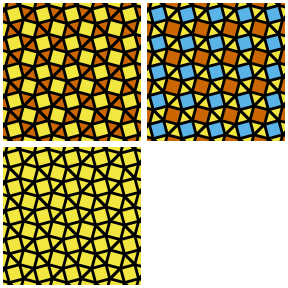## Truncated square tiling

We can achieve the two uniform colorings of a truncated square tiling using the “convex8” shape rotated 22.5 degrees. Adding in an extra (possibly alternating) “star8” shape results in a uniform tiling using star polygons.

# 2 color uniform truncated square tiling
pushViewport(viewport(x=0.25, y=0.75, width=0.5, height=0.5))
gp <- gpar(fill = red, col = NA)
grid.polygon(x_sq, y_sq, gp = gp)
gp <- gpar(fill = yellow, col = "black")
grid.pattern_regular_polygon(shape = "convex8", density = 1.082, rot = 22.5,
angle = 0, spacing = 0.3, gp = gp)
popViewport()

# 3 color uniform truncated square tiling
pushViewport(viewport(x=0.75, y=0.75, width=0.5, height=0.5))
gp <- gpar(fill = blue, col = NA)
grid.polygon(x_sq, y_sq, gp = gp)
gp <- gpar(fill = c(red, yellow), col = "black")
grid.pattern_regular_polygon(shape = "convex8", density = 1.082, rot = 22.5,
angle = 0, spacing = 0.3, gp = gp)
popViewport()

# 3 color uniform truncated square tiling with star
pushViewport(viewport(x=0.25, y=0.25, width=0.5, height=0.5))
gp <- gpar(fill = red, col = NA)
grid.polygon(x_sq, y_sq, gp = gp)
gp <- gpar(fill = yellow, col = "black")
grid.pattern_regular_polygon(shape = "convex8", density = 1.082, rot = 22.5,
angle = 0, spacing = 0.3, gp = gp)
gp <- gpar(fill = orange, col = "black")
scale <- star_scale(8, 60, external = TRUE)
grid.pattern_regular_polygon(shape = "star8", density = 1.082, rot = 22.5,
angle = 0, scale = scale, spacing = 0.3, gp = gp)
popViewport()

# 4 color uniform truncated square tiling with an alternating star
pushViewport(viewport(x=0.75, y=0.25, width=0.5, height=0.5))
gp <- gpar(fill = blue, col = NA)
grid.polygon(x_sq, y_sq, gp = gp)
gp <- gpar(fill = c(red, yellow), col = "black")
grid.pattern_regular_polygon(shape = "convex8", density = 1.082, rot = 22.5,
angle = 0, spacing = 0.3, gp = gp)
gp <- gpar(fill = orange, col = c("black"))
grid.pattern_regular_polygon(shape = c("null", "star8"),
density = 1.082, rot = 22.5,
angle = 0, scale = scale, spacing = 0.3, gp = gp)
popViewport()
add_borders()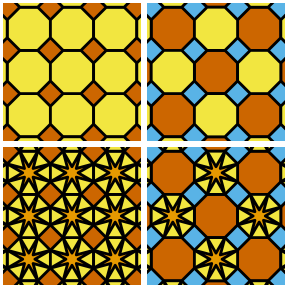## Truncated hexagonal tiling

We can achieve a truncated hexagonal tiling using the “convex12” shape with a 15 degree rotation in a “hex_circle” grid. The related “star12” variations are fun tilings as well.

# 2 color uniform truncated hexagonal tiling
grid.truncated_hexagonal_tiling <- function(..., shape = "convex12") {
scale <- star_scale(12, 60, external = TRUE)
grid.pattern_regular_polygon(..., shape = shape, density = 1.034, spacing = 0.3,
angle = 0, rot = 15, scale = scale,
grid = "hex_circle")
}
pushViewport(viewport(x=0.25, y=0.75, width=0.5, height=0.5))
gp <- gpar(fill = red, col = NA)
grid.polygon(x_sq, y_sq, gp = gp)
gp <- gpar(fill = yellow, col = "black")
grid.truncated_hexagonal_tiling(gp = gp)
popViewport()

# Add in a "convex12" star within
pushViewport(viewport(x=0.75, y=0.75, width=0.5, height=0.5))
gp <- gpar(fill = red, col = NA)
grid.polygon(x_sq, y_sq, gp = gp)
gp <- gpar(fill = yellow, col = "black")
grid.truncated_hexagonal_tiling(gp = gp)
gp <- gpar(fill = blue, col = "black")
grid.truncated_hexagonal_tiling(gp = gp, shape = "star12")
popViewport()

# Use a "star12" instead of a "convex12"
pushViewport(viewport(x=0.25, y=0.25, width=0.5, height=0.5))
gp <- gpar(fill = yellow, col = NA)
grid.polygon(x_sq, y_sq, gp = gp)
gp <- gpar(fill = blue, col = "black")
grid.truncated_hexagonal_tiling(gp = gp, shape = "star12")
popViewport()
add_borders()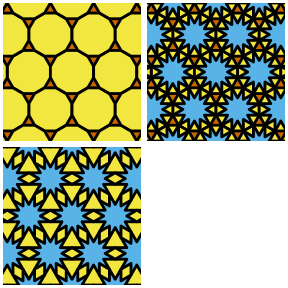## Trihexagonal tiling

We can achieve one of the uniform colorings of a trihexagonal tiling using the “convex6” shape with a “hex_circle” grid and rotating the shape 30 degrees.

There are topologically equivalent tilings using the “star6”, “star3”, and “convex3” shapes that also provides an interesting tiling effect.

grid.trihexagonal_tiling <- function(..., shape = "convex6", density = 1) {
grid.pattern_regular_polygon(..., shape = shape, density = density, grid = "hex_circle",
spacing = 0.2, rot = 30, angle = 0)
}
# 2 color uniform trihexagonal tiling
pushViewport(viewport(x=0.25, y=0.75, width=0.5, height=0.5))
grid.polygon(x_sq, y_sq, gp = gpar(fill = red, col = NA))
gp <- gpar(fill = yellow, col = "black")
grid.trihexagonal_tiling(gp = gp)
popViewport()

# 'star6' trihexagonal tiling
pushViewport(viewport(x=0.75, y=0.75, width=0.5, height=0.5))
grid.polygon(x_sq, y_sq, gp = gpar(fill = red, col = NA))
scale <- star_scale(6, 120, external = TRUE)
grid.trihexagonal_tiling(shape = "star6", scale = scale, gp = gp)
popViewport()

# 'star3' trihexagonal tiling
pushViewport(viewport(x=0.25, y=0.25, width=0.5, height=0.5))
grid.polygon(x_sq, y_sq, gp = gpar(fill = red, col = NA))
grid.trihexagonal_tiling(shape = "star3", scale = 0.2, density=1.65, gp = gp)
popViewport()

# 'convex3' trihexagonal tiling
pushViewport(viewport(x=0.75, y=0.25, width=0.5, height=0.5))
grid.polygon(x_sq, y_sq, gp = gpar(fill = red, col = NA))
grid.trihexagonal_tiling(shape = "convex3", density=1.33, gp = gp)
popViewport()
add_borders()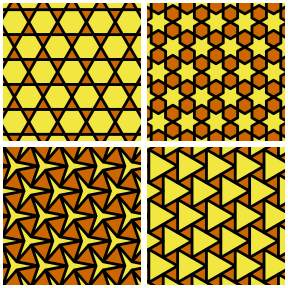## Snub trihexagonal tiling

We can achieve the one uniform colorings of a snub (tri)hexagonal tiling using a “star6” shape and a “convex6” shape.

grid.snub_trihexagonal <- function(..., gp_tri, gp_tri2 = gp_tri, gp_hex = gp_tri) {
scale_star <- star_scale(6, 60 + 60, external = TRUE)
grid.polygon(x_sq, y_sq, gp = gp_tri)
grid.pattern_regular_polygon(shape = "star6", scale = scale_star,
grid = "hex_circle",
angle = 0, rot = 19, spacing = 0.2,
density = 1.305, gp = gp_tri2)
grid.pattern_regular_polygon(shape = "convex6", grid = "hex_circle",
angle = 0, rot = 19 + 30, spacing = 0.2,
density = scale_star * 1.305, gp = gp_hex)
}

# 3 color uniform snub square tiling
pushViewport(viewport(x=0.25, y=0.75, width=0.5, height=0.5))
gp_y <- gpar(fill = yellow, col = "black")
gp_r <- gpar(fill = red, col = "black")
gp_b <- gpar(fill = blue, col = "black")
grid.snub_trihexagonal(gp_tri = gp_b, gp_tri2 = gp_r, gp_hex = gp_y)
popViewport()

# 2 color snub trihexagonal tiling
pushViewport(viewport(x=0.75, y=0.75, width=0.5, height=0.5))
grid.snub_trihexagonal(gp_tri = gp_y, gp_hex = gp_r)
popViewport()

# 1 color snub trihexagonal tiling
pushViewport(viewport(x=0.25, y=0.25, width=0.5, height=0.5))
grid.snub_trihexagonal(gp_tri = gp_y)
popViewport()

pushViewport(viewport(x=0.75, y=0.25, width=0.5, height=0.5))
popViewport()
add_borders()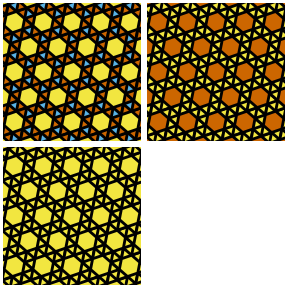## Truncated trihexagonal

By carefully using grid.pattern_stripe() it is possible to achieve the truncated trihexagonal tiling.

# truncated trihexagonal
pushViewport(viewport(x=0.25, y=0.75, width=0.5, height=0.5))
grid.polygon(x_sq, y_sq, gp = gpar(fill = yellow, col = NA))
gp <- gpar(fill = red, col = "black")
grid.pattern_stripe(grid = "hex_circle", density = 0.42, spacing = 0.3,
angle = 0, gp = gp)
grid.pattern_stripe(grid = "hex_circle", density = 0.42, spacing = 0.3,
angle = -60, gp = gp)
grid.pattern_stripe(grid = "hex_circle", density = 0.42, spacing = 0.3,
angle = 60, gp = gp)
gp <- gpar(fill = blue, col = "black")
grid.pattern_regular_polygon(shape = "convex6", grid = "hex_circle",
density = 0.75, spacing = 0.3, angle = 0, gp = gp)
popViewport()

# truncated trihexagonal with 5 colors
pushViewport(viewport(x=0.75, y=0.75, width=0.5, height=0.5))
grid.polygon(x_sq, y_sq, gp = gpar(fill = green, col = NA))
gp <- gpar(fill = orange, col = "black")
grid.pattern_stripe(grid = "hex_circle", density = 0.42, spacing = 0.3,
angle = 0, gp = gp)
grid.pattern_stripe(grid = "hex_circle", density = 0.42, spacing = 0.3,
angle = -60, gp = gp)
grid.pattern_stripe(grid = "hex_circle", density = 0.42, spacing = 0.3,
angle = 60, gp = gp)
gp <- gpar(fill = c(yellow, red, blue), col = "black")
grid.pattern_regular_polygon(shape = "convex6", grid = "hex_circle",
density = 0.75, spacing = 0.3, angle = 0, gp = gp)
popViewport()
pushViewport(viewport(x=0.25, y=0.25, width=0.5, height=0.5))
popViewport()
pushViewport(viewport(x=0.75, y=0.25, width=0.5, height=0.5))
popViewport()
add_borders()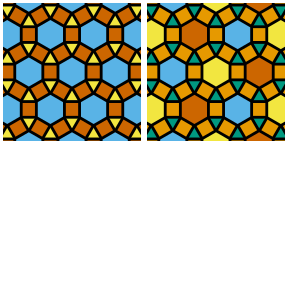## Rhombitrihexagonal tiling

By carefully using grid.pattern_stripe() it is also possible to achieve the rhombitrihexagonal tiling. There are also variant tilings where we add in “star12” polygons.

# rhombitrihexagonal
pushViewport(viewport(x=0.25, y=0.75, width=0.5, height=0.5))
grid.polygon(x_sq, y_sq, gp = gpar(fill = yellow, col = NA))
gp <- gpar(fill = red, col = "black")
grid.pattern_stripe(grid = "hex_circle", density = 0.25, spacing = 0.3,
angle = 0, gp = gp)
grid.pattern_stripe(grid = "hex_circle", density = 0.25, spacing = 0.3,
angle = -60, gp = gp)
grid.pattern_stripe(grid = "hex_circle", density = 0.25, spacing = 0.3,
angle = 60, gp = gp)
gp <- gpar(fill = blue, col = "black")
grid.pattern_regular_polygon(shape = "convex12", grid = "hex_circle", rot = 15,
density = 0.82, spacing = 0.3, angle = 0, gp = gp)
popViewport()

# rhombitrihexagonal with 5 colors
pushViewport(viewport(x=0.75, y=0.75, width=0.5, height=0.5))
grid.polygon(x_sq, y_sq, gp = gpar(fill = green, col = NA))
gp <- gpar(fill = red, col = "black")
grid.pattern_stripe(grid = "hex_circle", density = 0.25, spacing = 0.3,
angle = 0, gp = gp)
gp <- gpar(fill = yellow, col = "black")
grid.pattern_stripe(grid = "hex_circle", density = 0.25, spacing = 0.3,
angle = -60, gp = gp)
gp <- gpar(fill = blue, col = "black")
grid.pattern_stripe(grid = "hex_circle", density = 0.25, spacing = 0.3,
angle = 60, gp = gp)
gp <- gpar(fill = orange, col = "black")
grid.pattern_regular_polygon(shape = "convex12", grid = "hex_circle", rot = 15,
density = 0.82, spacing = 0.3, angle = 0, gp = gp)
popViewport()

# 3.4.6.3.12*_{π/6} (rhombitrihexagonal plus star)
pushViewport(viewport(x=0.25, y=0.25, width=0.5, height=0.5))
grid.polygon(x_sq, y_sq, gp = gpar(fill = yellow, col = NA))
gp <- gpar(fill = red, col = "black")
grid.pattern_stripe(grid = "hex_circle", density = 0.25, spacing = 0.3,
angle = 0, gp = gp)
grid.pattern_stripe(grid = "hex_circle", density = 0.25, spacing = 0.3,
angle = -60, gp = gp)
grid.pattern_stripe(grid = "hex_circle", density = 0.25, spacing = 0.3,
angle = 60, gp = gp)
gp <- gpar(fill = blue, col = "black")
grid.pattern_regular_polygon(shape = "convex12", grid = "hex_circle", rot = 15,
density = 0.82, spacing = 0.3, angle = 0, gp = gp)
gp <- gpar(fill = green, col = "black")
scale <- star_scale(12, 30)
grid.pattern_regular_polygon(shape = "star12", grid = "hex_circle", rot = 15,
density = 0.82, spacing = 0.3, angle = 0, gp = gp,
scale = scale)
popViewport()

pushViewport(viewport(x=0.75, y=0.25, width=0.5, height=0.5))
popViewport()
add_borders()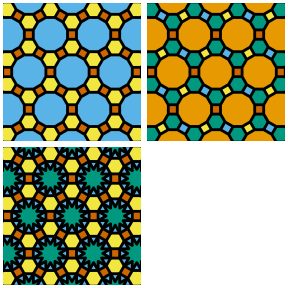## Additional uniform tilings with star polygons

Some additional uniform tilings with star polygons not shown above.

# 12.12.4_{π/3}
pushViewport(viewport(x=0.25, y=0.75, width=0.5, height=0.5))
grid.polygon(x_sq, y_sq, gp = gpar(fill = red, col = NA))
gp <- gpar(fill = c(yellow, blue), col = "black")
grid.pattern_regular_polygon(shape="convex12", rot=15, density=1.04,
angle=0, gp = gp, spacing=0.3)
popViewport()

# (12.3_{π/6})^2
pushViewport(viewport(x=0.75, y=0.75, width=0.5, height=0.5))
grid.polygon(x_sq, y_sq, gp = gpar(fill = red, col = NA))
gp <- gpar(fill = c(yellow), col = "black")
grid.pattern_regular_polygon(shape="convex12", density=1, grid = "hex_circle",
angle=0, gp = gp, spacing=0.4)
popViewport()

# 4.4*_α.4**_α
pushViewport(viewport(x=0.25, y=0.25, width=0.5, height=0.5))
grid.polygon(x_sq, y_sq, gp = gpar(fill = red, col = NA))
gp <- gpar(fill = c(yellow, blue), col = "black")
grid.pattern_regular_polygon(shape="star4", density=1.64, angle=0, scale=0.27,
rot=-9.0, spacing=0.3, gp = gp)
popViewport()

# 3.3.8*.4**.8*
pushViewport(viewport(x=0.75, y=0.25, width=0.5, height=0.5))
sp <- 0.35
gp <- gpar(fill = red, col = "black")
grid.pattern_regular_polygon(shape = "square", density = 1, angle = 0,
spacing = sp, gp = gp)
gp <- gpar(fill = yellow, col = "black")
grid.pattern_regular_polygon(shape = "star4", density = 1.08, rot = 45,
xoffset = sp / 2, yoffset = sp / 2,
angle = 0, scale = 0.56, spacing = sp, gp = gp)
gp <- gpar(fill = blue, col = "black")
scale <- star_scale(8, 15)
grid.pattern_regular_polygon(shape = "star8", density = 1.082, rot = 22.5,
angle = 0, scale = 0.30, spacing = sp, gp = gp)
popViewport()
add_borders()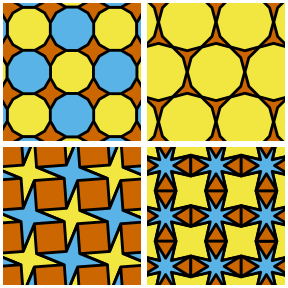# 3.6*.6*
pushViewport(viewport(x=0.25, y=0.75, width=0.5, height=0.5))
grid.polygon(x_sq, y_sq, gp = gpar(fill = red, col = NA))
gp <- gpar(fill = yellow, col = "black")
grid.pattern_regular_polygon(shape="star6", density=1.25, angle=0, scale=0.4, rot=-21.9,
spacing=0.3, grid="hex", gp=gp)
popViewport()

# 4.6.4*.6
pushViewport(viewport(x=0.75, y=0.75, width=0.5, height=0.5))
grid.polygon(x_sq, y_sq, gp = gpar(fill = yellow, col = NA))
gp <- gpar(fill = c(blue, red), col = "black")
scale <- star_scale(4, 120, external = TRUE)
grid.pattern_regular_polygon(shape=c("star4", "convex4"), density=c(1.2, 0.8),
scale = scale,
spacing = 0.2, gp = gp, angle=45)
popViewport()

# 9.3.9.3*_{π/9}
pushViewport(viewport(x=0.25, y=0.25, width=0.5, height=0.5))
grid.polygon(x_sq, y_sq, gp = gpar(fill = red, col = NA))
gp <- gpar(fill = blue, col = NA)
grid.pattern_stripe(spacing = 0.4, density = 0.35, angle = 0,
grid = "hex_circle", gp = gp, yoffset = 0.096)
gp <- gpar(fill = yellow, col = "black")
grid.pattern_regular_polygon(shape = "convex9", density = 1.01, angle = 0,
grid = "hex_circle", spacing = 0.4, gp = gp)
popViewport()

# 8.4*.8.4*
pushViewport(viewport(x=0.75, y=0.25, width=0.5, height=0.5))
grid.polygon(x_sq, y_sq, gp = gpar(fill = red, col = NA))
gp <- gpar(fill = yellow, col = "black")
grid.pattern_regular_polygon(shape = c("convex8", "null"), density = 1.41,
angle = 0, spacing = 0.2, gp = gp)
popViewport()
add_borders()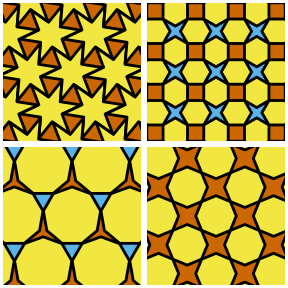# Non-uniform tilings

## Herringbone tiling

We can achieve a herringbone tiling by using grid.pattern_weave() and particular “twill” weaves of the subtype “n/n(1)”.

grid.herringbone_tiling <- function(..., subtype = "2/2(1)") {
grid.pattern_weave(..., type = "twill", subtype = subtype, density = 1,
spacing = 0.1, angle = 45)
}
# 1 color 2:1 herringbone
pushViewport(viewport(x=0.25, y=0.75, width=0.5, height=0.5))
gp <- gpar(fill = yellow, col = "black")
grid.herringbone_tiling(gp = gp)
popViewport()

# 2 color 2:1 herringbone
pushViewport(viewport(x=0.75, y=0.75, width=0.5, height=0.5))
gp <- gpar(fill = yellow, col = "black")
grid.herringbone_tiling(gp = gp, fill2 = red)
popViewport()

# 1 color 3:1 herringbone
pushViewport(viewport(x=0.25, y=0.25, width=0.5, height=0.5))
gp <- gpar(fill = yellow, col = "black")
grid.herringbone_tiling(gp = gp, subtype = "3/3(1)")
popViewport()

# 2 color 3:1 herringbone
pushViewport(viewport(x=0.75, y=0.25, width=0.5, height=0.5))
gp <- gpar(fill = yellow, col = "black")
grid.herringbone_tiling(gp = gp, fill2 = red, subtype = "3/3(1)")
popViewport()
add_borders()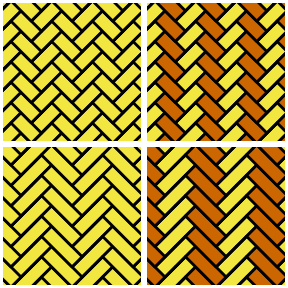## Pythagorean tiling

We can get a Pythagorean tiling by setting a background color and rotating “convex4” shapes

grid.pythagorean_tiling <- function(...) {
grid.pattern_regular_polygon(..., shape = "convex4", density = 1.222,
rot = 15, spacing = 0.2, angle = -15)
}
# 1 color Pythagorean tiling
pushViewport(viewport(x=0.25, y=0.75, width=0.5, height=0.5))
grid.polygon(x_sq, y_sq, gp = gpar(fill = yellow, col = NA))
gp <- gpar(fill = yellow, col = "black")
grid.pythagorean_tiling(gp = gp)
popViewport()

# 2 color Pythagorean tiling
pushViewport(viewport(x=0.75, y=0.75, width=0.5, height=0.5))
grid.polygon(x_sq, y_sq, gp = gpar(fill = yellow, col = NA))
gp <- gpar(fill = red, col = "black")
grid.pythagorean_tiling(gp = gp)
popViewport()

# 3 color Pythagorean tiling
pushViewport(viewport(x=0.25, y=0.25, width=0.5, height=0.5))
grid.polygon(x_sq, y_sq, gp = gpar(fill = yellow, col = NA))
gp <- gpar(fill = c(blue, red), col = "black")
grid.pythagorean_tiling(gp = gp)
popViewport()

# 4 color Pythagorean tiling
pushViewport(viewport(x=0.75, y=0.25, width=0.5, height=0.5))
grid.polygon(x_sq, y_sq, gp = gpar(fill = yellow, col = NA))
gp <- gpar(fill = c(red, blue, green), col = "black")
grid.pythagorean_tiling(gp = gp)
popViewport()
add_borders()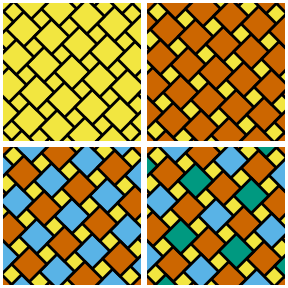## Rhombille tiling

We can get a single-color Rhombille tiling by using the “convex6” and a very narrow “star3” shape:

gp <- gpar(fill = yellow, col = "black")
grid.pattern_regular_polygon(shape = "convex6", density = 1, angle = 0,
grid = "hex", gp = gp, spacing = 0.1)
gp <- gpar(fill = NA, col = "black")
grid.pattern_regular_polygon(shape = "star3", density = 1, angle = 0, rot = 60,
grid = "hex", gp = gp, scale = 0.001, spacing = 0.1)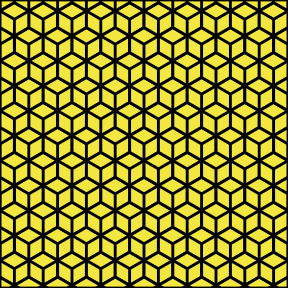## Tetrakis square tiling

We can get a single-color Tetrakis square tiling by using the “square” and a very narrow “star4” shape:

gp <- gpar(fill = yellow, col = "black")
grid.pattern_regular_polygon(shape = "square", density = 1, angle = 0,
gp = gp, spacing = 0.1)
gp <- gpar(fill = NA, col = "black")
grid.pattern_regular_polygon(shape = "star4", density = 1.37, angle = 0, rot = 45,
gp = gp, scale = 0.001, spacing = 0.2,
xoffset = 0.05, yoffset = 0.05)